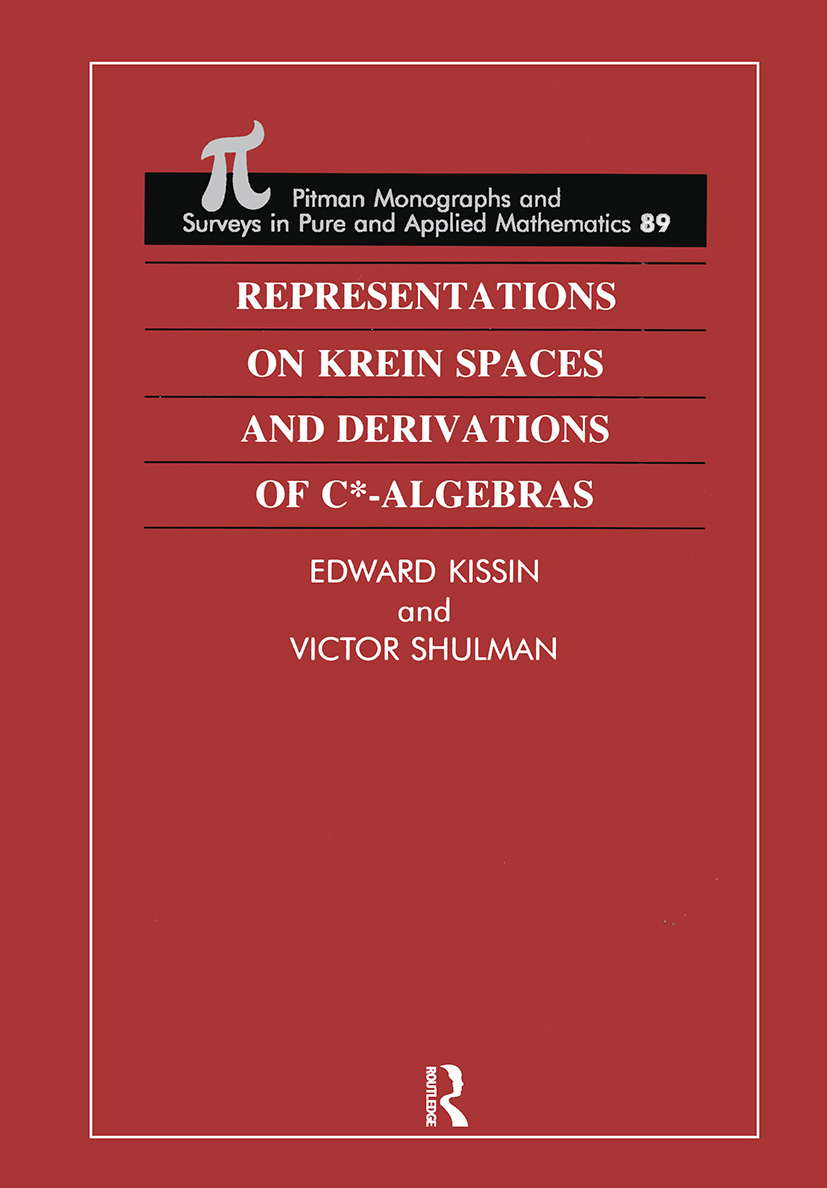# Representations on Krein Spaces [Hot] and Derivations of C*-Algebras

## 1st Edition

Chapman and Hall/CRC

616 pages

Hardback: 9780582231573
pub: 1997-10-03
Currently out of stock
\$335.00
x

FREE Standard Shipping!

### Description

This text provides a comprehensive treatment of representations on indefinite metric spaces, and their applications to the theory of *-derivations of C*-algebras.

The book consists of two parts. The first studies the geometry of indefinite metric spaces (Krein and (Pi)(kappa)-spaces) and describes the theory of J-symmetric operator algebras and representations of *-algebras and groups on these spaces in a systematic form. For representations on (Pi)(kappa)-spaces, many significant new results are obtained; this establishes a possible approach to the general theory of representations.

In the second part, different techniques of the theory of J-symmetric representations on Krein spaces are applied to the theory of *-derivations of C*-algebras implemented by skew-symmetric and dissipative operators. Various results are obtained, which establish a link between the deficiency indices of skew-symmetric operators implementing *-derivations of C*-algebras and dimensions of representations of these algebras. The problem of isomorphism of skew-symmetric operators is also touched upon.

Numerous properties of the domains of *-derivations are investigated. These domains constitute an important subclass of differentiable Banach *-algebras, that is dense *-subalgebras of C*-algebras with properties in many respects similar to the properties of algebras of differentiable functions. The Weyl operator commutation relations are examined in the general context of *-derivations of C*-algebras. Powersí and Arvesonís indices of one-parameter semigroups of *-endomorphisms of the algebra B are considered, and various notions of the index of a *-derivation are introduced and studied.

Application of the theory of J-symmetric representations on Krein spaces to the theory of *-derivations of C*-algebras is a new research area of growing interest and there are many exciting advances to be made in this field. The book covers a fairly large and complex body of material, and will serve as a stimulus to further research activity in this area.

Preface

Background

Spaces with Indefinite Metric

J-Symmetric Operator Algebras and Representations on Krein Spaces

Non-Degenerate J-Symmetric Operator Algebras on (Pi)(kappa)-Spaces

J-Symmetric Operator Algebras on (Pi)1-Spaces

Representations of *-Algebras and Groups on (Pi)(kappa)-Spaces

Derivations of C*-Algebras Implemented by Skew-Symmetric Operators

Bibliography

Index